# Graphs of piecewise linear functions

##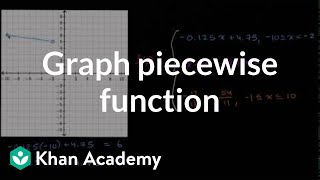By Khan Academy

Sal graphs a piecewise function given its formula. In this case, the function consists of three separate lines.# Graphs of piecewise linear functions

##By Khan Academy

Sal explains what a piecewise function is, and finds the formula of a piecewise function given its graph.# Graphs Of Square Root Functions

##By Khan Academy

This Khan Academy video offers more information on graphing square root functions and contains a few sample problems with a step-by-step walkthrough on graphing these functions.# Interpreting graphs of linear and nonlinear functions

##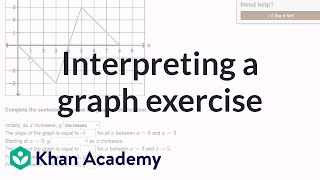By Khan Academy

Interpreting graphs of linear and nonlinear functions# Graphing Cube Root Functions

##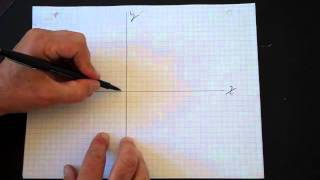By drmikedcook

This video explains explains how to graph cube root functions.# Match graphs of rational functions to their formula

##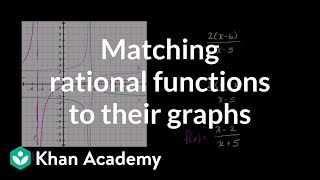By Khan Academy

Sal matches three graphs of rational functions to three formulas of such functions by considering asymptotes and intercepts.# Graphing a family of cubic functions

##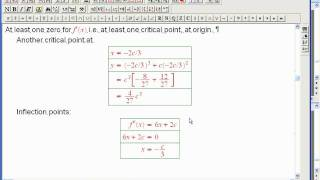By David Metzler

Using calculus to analyze and graph a family of cubic functions in conjunction with technology# Quickly Graph Absolute Value Functions

##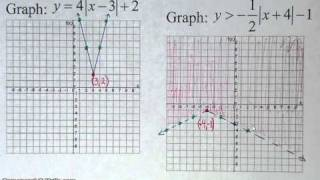By WOWmath.org

YouTube presents Quickly Graph Absolute Value Functions, an educational video resource on math.# Graph Functions and Relations

##By MathPlanetVideos

This video from MathPlanet provides an overview of algebraic concepts related to graphing functions and relations.# Graph Functions and Relations

##By MathPlanetVideos

This video from MathPlanet provides an overview of algebraic concepts related to graphing functions and relations.# Graphs of piecewise linear functions

##By Khan Academy

Sal explains what a piecewise function is, and finds the formula of a piecewise function given its graph.# Graphs of piecewise linear functions

##By Khan Academy

Sal explains what a piecewise function is, and finds the formula of a piecewise function given its graph.# Graphs of piecewise linear functions

##By Khan Academy

Sal graphs a piecewise function given its formula. In this case, the function consists of three separate lines.# Graphs of piecewise linear functions

##By Khan Academy

Sal graphs a piecewise function given its formula. In this case, the function consists of three separate lines.# Graphing Absolute Value Functions Pt III

##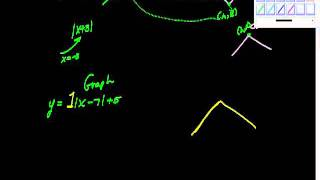By charlie Lindelof

YouTube presents Graphing Absolute Value Functions Pt III, an educational video resource on mathematics.# Graphing Quadratic Functions - Example 1

##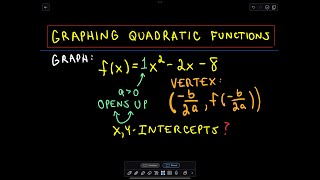By PatrickJMT

Watch this video to learn how to graph a quadratic function of the form ax2 +bx + c, where a, b, and c are constants (and a≠0). All functions of this form describe the graph of a parabola (u-shape) that either opens upward or downward. To graph a quadratic function: Consider the leading coefficient (the value of a) to determine if the parabola opens upward (postive a) or downward (negative a) Calculate the vertex of the parabola from a and b. The vertex is the highest or lowest point of the parabola. Find the x-intercept(s) (zeros) Find the y-intercept. (Found by plugging x=0 into the function).# Math 8 Honors: Chapter 12.2- Graphing Rational Functions

##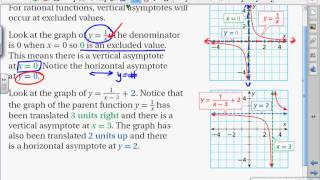By Megan Frantz

Graphing Rational Functions# Using the x- and y- Intercepts to Graph a Linear Function

##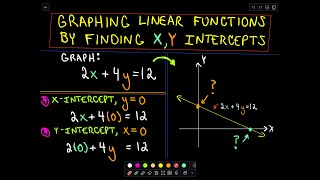By PatrickJMT

This video shows us how to use two important features of a linear function, the x- and y- intercepts, to graph the function. Remember that we only need two points to determine a line, and often, the intercepts are extremely easy to find.# Graphing Transformations of the Cube Root Function

##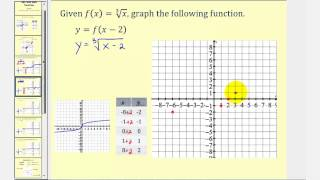By Mathispower4u

Graphing Transformations of the Cube Root Function# How to Graph an Exponential Function - College Algebra Tips

##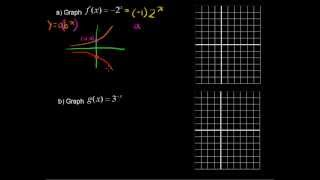By StraighterLine

Review how to Graph an Exponential Function in this College Algebra tutorial. Watch and learn now! Then take an online College Algebra course at StraighterLine for college